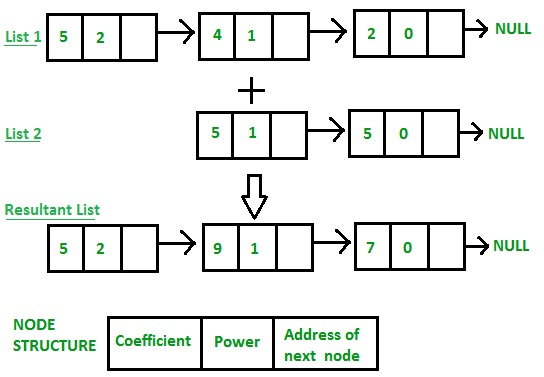• Last Updated : 06 Jan, 2022

Given two polynomial numbers represented by a linked list. Write a function that add these lists means add the coefficients who have same variable powers.
Example:

```Input:
1st number = 5x2 + 4x1 + 2x0
2nd number = -5x1 - 5x0
Output:
5x2-1x1-3x0
Input:
1st number = 5x3 + 4x2 + 2x0
2nd number = 5x^1 - 5x^0
Output:
5x3 + 4x2 + 5x1 - 3x0```## Java

 `import` `java.io.*; ` `import` `java.util.Scanner; ` `class` `Polynomial  ` `{ ` `    ``public` `static` `Node addPolynomial(Node p1,  ` `                                     ``Node p2) ` `    ``{ ` `        ``Node a = p1, b = p2,  ` `             ``newHead = ``new` `Node(``0``, ``0``), ` `             ``c = newHead; ` ` `  `        ``while` `(a != ``null` `|| b != ``null``)  ` `        ``{ ` `            ``if` `(a == ``null``)  ` `            ``{ ` `                ``c.next = b; ` `                ``break``; ` `            ``} ` `            ``else` `if` `(b == ``null``)  ` `            ``{ ` `                ``c.next = a; ` `                ``break``; ` `            ``} ` ` `  `            ``else` `if` `(a.pow == b.pow)  ` `            ``{ ` `                ``c.next = ``new` `Node(a.coeff +  ` `                                  ``b.coeff, a.pow); ` `                ``a = a.next; ` `                ``b = b.next; ` `            ``} ` ` `  `            ``else` `if` `(a.pow > b.pow)  ` `            ``{ ` `                ``c.next = ``new` `Node(a.coeff,  ` `                                  ``a.pow); ` `                ``a = a.next; ` `            ``} ` ` `  `            ``else` `if` `(a.pow < b.pow)  ` `            ``{ ` `                ``c.next = ``new` `Node(b.coeff,  ` `                                  ``b.pow); ` `                ``b = b.next; ` `            ``} ` `            ``c = c.next; ` `        ``} ` `        ``return` `newHead.next; ` `    ``} ` `} ` ` `  `// Utilities for Linked List  ` `// Nodes ` `class` `Node  ` `{ ` `    ``int` `coeff; ` `    ``int` `pow; ` `    ``Node next; ` `    ``Node(``int` `a, ``int` `b) ` `    ``{ ` `        ``coeff = a; ` `        ``pow = b; ` `        ``next = ``null``; ` `    ``} ` `} ` ` `  `// Linked List main class ` `class` `LinkedList  ` `{   ` `    ``public` `static` `void` `main(String args[]) ` `    ``{ ` `        ``Node start1 = ``null``, cur1 = ``null``,  ` `             ``start2 = ``null``, cur2 = ``null``; ` `        ``int``[] list1_coeff = {``5``, ``4``, ``2``}; ` `        ``int``[] list1_pow = {``2``, ``1``, ``0``}; ` `        ``int` `n = list1_coeff.length; ` ` `  `        ``int` `i = ``0``; ` `        ``while` `(n-- > ``0``)  ` `        ``{ ` `            ``int` `a = list1_coeff[i]; ` `            ``int` `b = list1_pow[i]; ` `            ``Node ptr = ``new` `Node(a, b); ` `            ``if` `(start1 == ``null``)  ` `            ``{ ` `                ``start1 = ptr; ` `                ``cur1 = ptr; ` `            ``} ` `            ``else`  `            ``{ ` `                ``cur1.next = ptr; ` `                ``cur1 = ptr; ` `            ``} ` `            ``i++; ` `        ``} ` ` `  `        ``int``[] list2_coeff = {-``5``, -``5``}; ` `        ``int``[] list2_pow = {``1``, ``0``}; ` `        ``n = list2_coeff.length; ` ` `  `        ``i = ``0``; ` `        ``while` `(n-- > ``0``)  ` `        ``{ ` `            ``int` `a = list2_coeff[i]; ` `            ``int` `b = list2_pow[i]; ` ` `  `            ``Node ptr = ``new` `Node(a, b); ` ` `  `            ``if` `(start2 == ``null``)  ` `            ``{ ` `                ``start2 = ptr; ` `                ``cur2 = ptr; ` `            ``} ` `            ``else`  `            ``{ ` `                ``cur2.next = ptr; ` `                ``cur2 = ptr; ` `            ``} ` `            ``i++; ` `        ``} ` ` `  `        ``Polynomial obj = ``new` `Polynomial(); ` `        ``Node sum = obj.addPolynomial(start1,  ` `                                     ``start2); ` `        ``Node trav = sum; ` `        ``while` `(trav != ``null``)  ` `        ``{ ` `            ``System.out.print(trav.coeff +  ` `                             ``"x^"` `+ trav.pow); ` `            ``if` `(trav.next != ``null``) ` `                ``System.out.print(``" + "``); ` `            ``trav = trav.next; ` `        ``} ` `        ``System.out.println(); ` `    ``} ` `}`

Output:

```1st Number: 5x^2+4x^1+2x^0
2nd Number: -5x^1-5x^0